The plot_tox_boxplots function creates a set of boxplots representing EAR values computed with the get_chemical_summary function, and dependent on the choice of several input options. See "Summarizing the data" in the Introduction vignette: vignette("Introduction", package = "toxEval"). for a description of how the EAR values are computed, aggregated, and summarized. Choosing "Chemical Class" in the category argument will generate separate boxplots for each unique class. "Chemical" will generate boxplots for each individual chemical, and "Biological" will generate boxplots for each group in the selected ToxCast annotation.

## Usage

plot_chemical_boxplots(
chemical_summary,
...,
manual_remove = NULL,
mean_logic = FALSE,
sum_logic = TRUE,
plot_ND = TRUE,
font_size = NA,
title = NA,
x_label = NA,
palette = NA,
hit_threshold = NA
)

plot_tox_boxplots(
chemical_summary,
category = "Biological",
manual_remove = NULL,
mean_logic = FALSE,
sum_logic = TRUE,
plot_ND = TRUE,
font_size = NA,
title = NA,
x_label = NA,
palette = NA,
hit_threshold = NA
)

## Arguments

chemical_summary

Data frame from get_chemical_summary.

...

Additional group_by arguments. This can be handy for creating facet graphs.

manual_remove

Vector of categories to remove.

mean_logic

Logical. TRUE displays the mean sample from each site, FALSE displays the maximum sample from each site.

sum_logic

Logical. TRUE sums the EARs in a specified grouping, FALSE does not. FALSE may be better for traditional benchmarks as opposed to ToxCast benchmarks.

plot_ND

Logical. Whether or not to plot "Biological" groupings, "Chemical Class" groupings, or "Chemical" that do not have any detections.

font_size

Numeric value to adjust the axis font size.

title

Character title for plot. Default is NA which produces no title.

x_label

Character for x label. Default is NA which produces an automatic label.

palette

Vector of color palette for boxplot fill. Can be a named vector to specify specific colors for specific categories.

hit_threshold

Numeric threshold defining a "hit".

category

Character. Either "Biological", "Chemical Class", or "Chemical".

## Details

It is also possible to display a threshold line using the hit_threshold argument. The graph will then include the number of sites with detections, the threshold line, and the number of "hits" indicating how many sites that have EAR values exceeding the hit_threshold.

The graph shows a slightly different result for a single site. For a single site graph, the number of chemicals that were detected and have associated endpoint ACCs represented are displayed.

The functions plot_tox_boxplots and graph_chem_data are functions that perform the statistical calculations to create the plot. graph_chem_data is specific to the "Chemical" plot, and plot_tox_boxplots is for "Biological" and "Chemical Class".

Box plots are standard Tukey representations. See "Box plot details" in the Basic Workflow vignette: vignette("basicWorkflow", package = "toxEval") for more information.

## Examples

# This is the example workflow:
path_to_tox <- system.file("extdata", package = "toxEval")
file_name <- "OWC_data_fromSup.xlsx"

full_path <- file.path(path_to_tox, file_name)

tox_list <- create_toxEval(full_path)
ACC <- get_ACC(tox_list$chem_info$CAS)
ACC <- remove_flags(ACC)

cleaned_ep <- clean_endPoint_info(end_point_info)
filtered_ep <- filter_groups(cleaned_ep)
chemical_summary <- get_chemical_summary(tox_list, ACC, filtered_ep)
plot_tox_boxplots(chemical_summary, "Biological")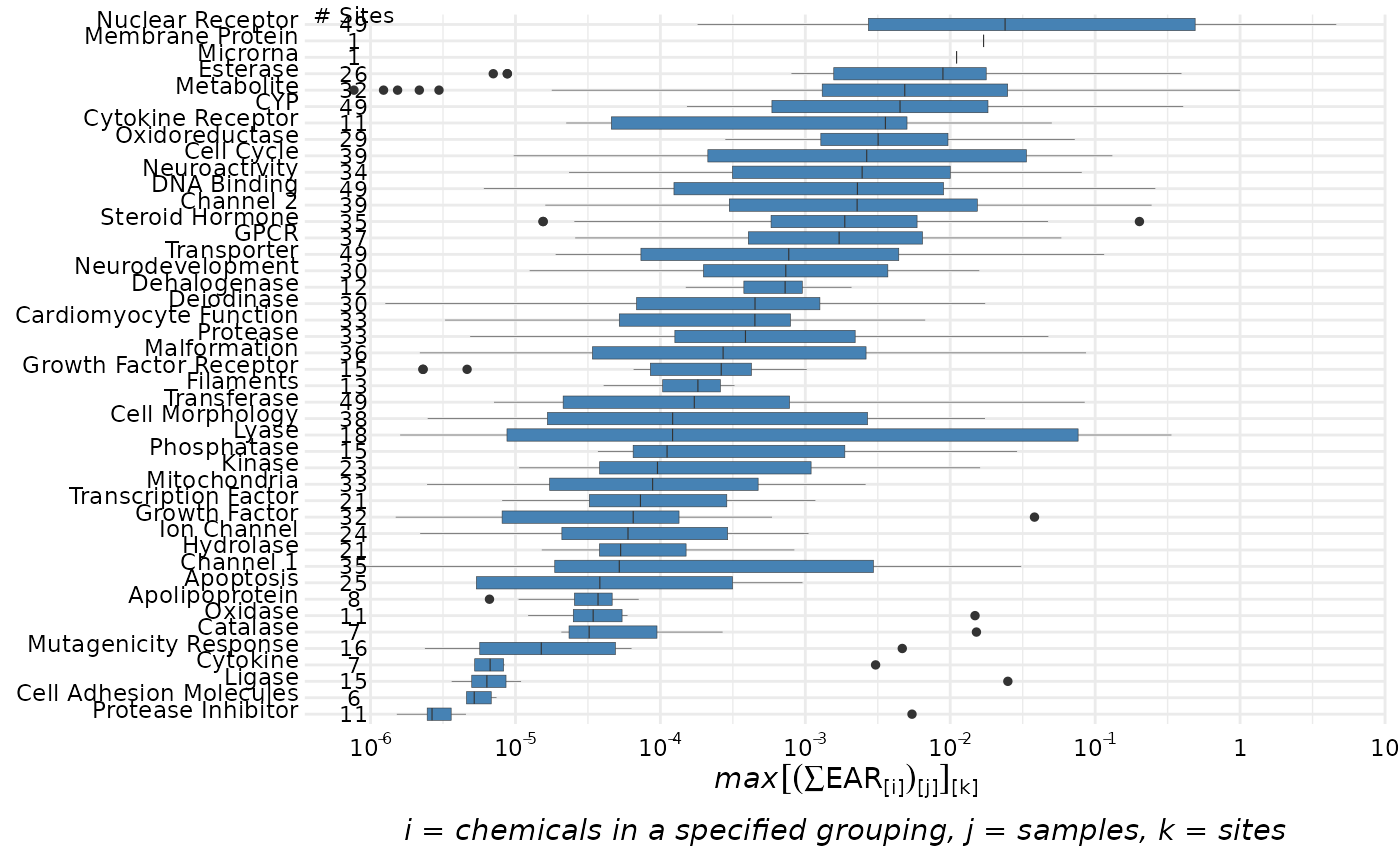# \donttest{
plot_tox_boxplots(chemical_summary, "Chemical Class")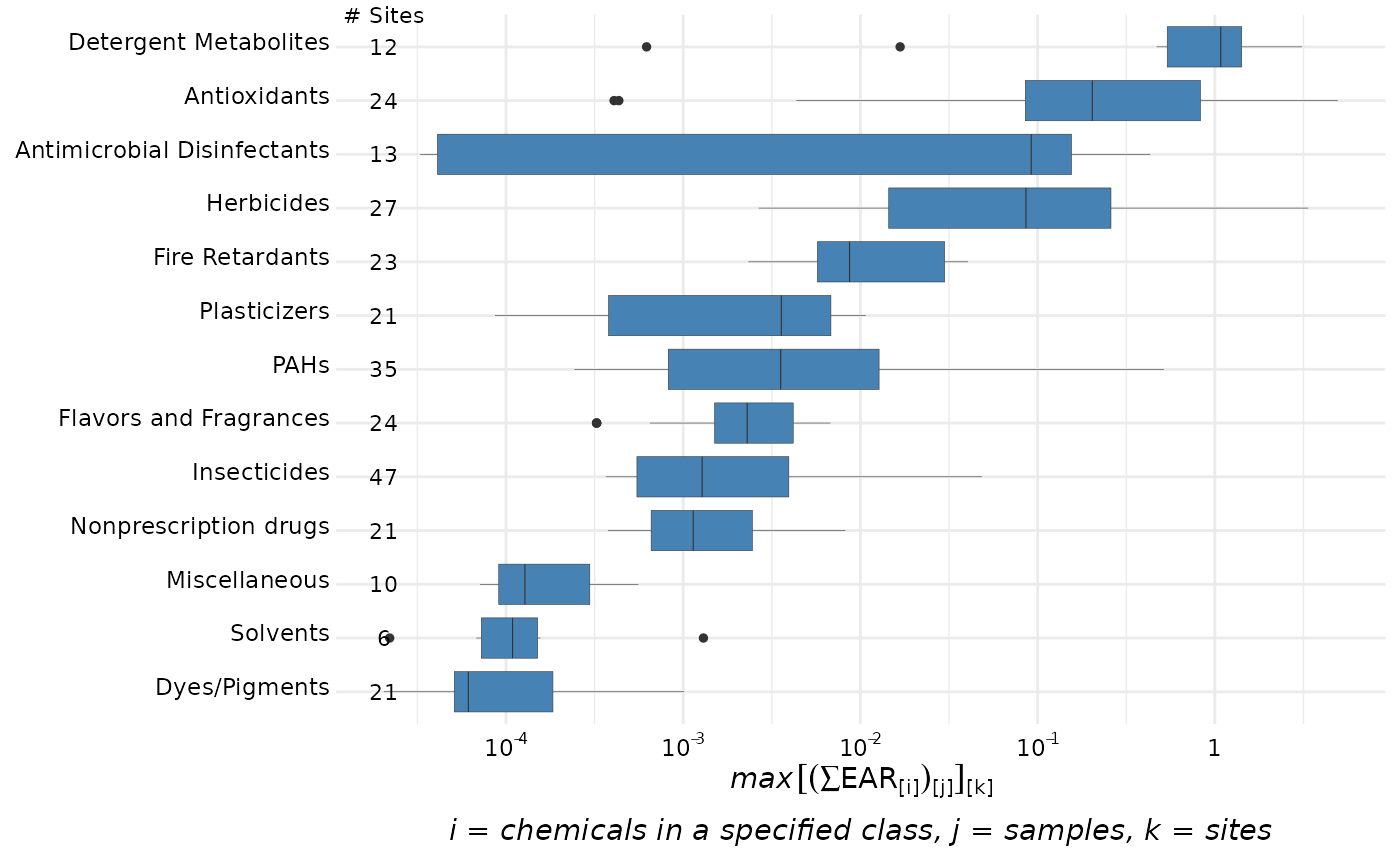plot_tox_boxplots(chemical_summary, "Chemical")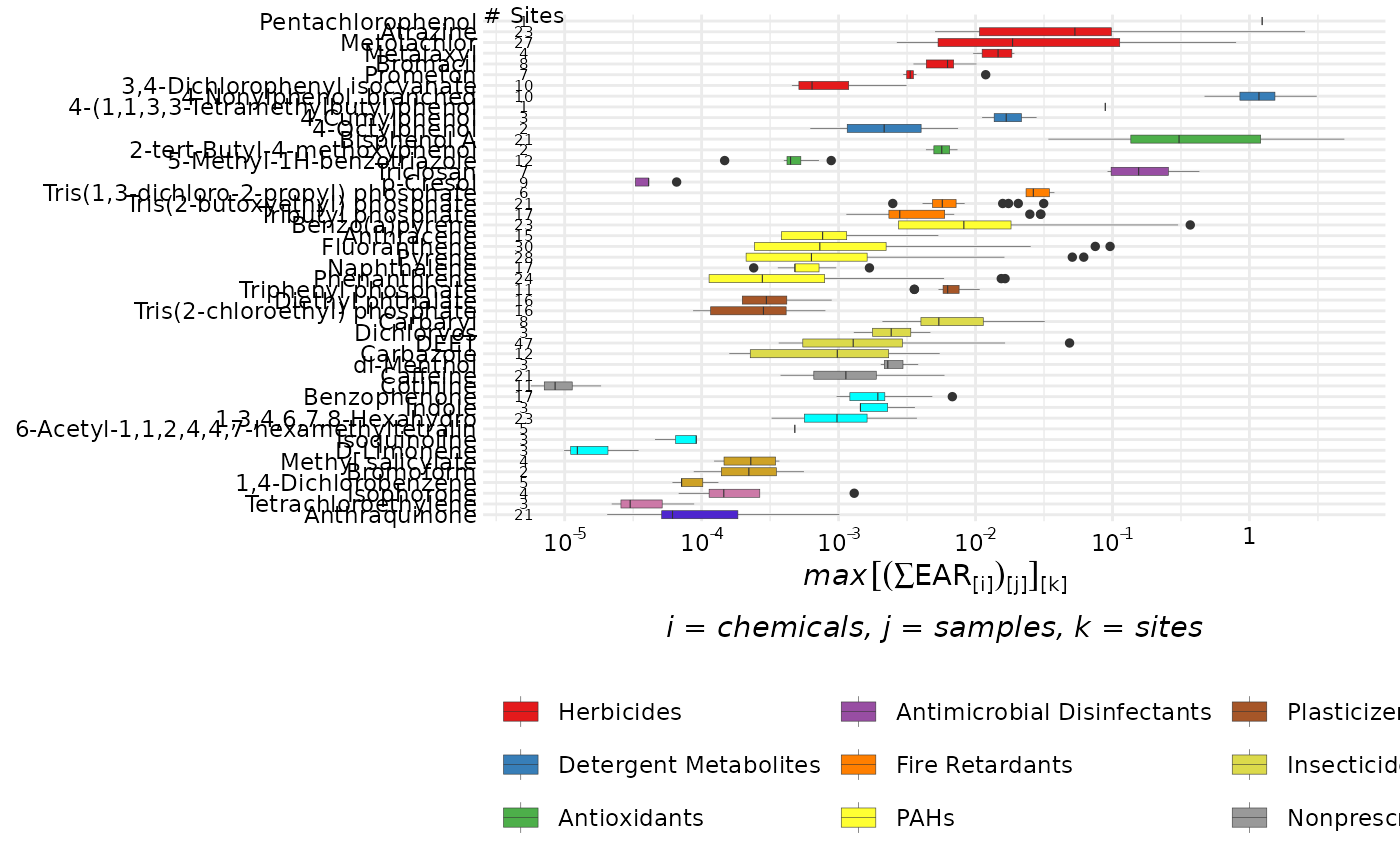cbPalette <- c(
"#999999", "#E69F00", "#56B4E9", "#009E73", "#F0E442",
"#0072B2", "#D55E00", "#CC79A7"
)
graphData <- tox_boxplot_data(
chemical_summary = chemical_summary,
category = "Biological"
)
cbValues <- colorRampPalette(cbPalette)(length(levels(graphData$category))) names(cbValues) <- levels(graphData$category)

plot_tox_boxplots(chemical_summary,
hit_threshold = 0.1,
category = "Biological",
palette = cbValues,
title = "Maximum EAR per site, grouped by biological activity groupings"
)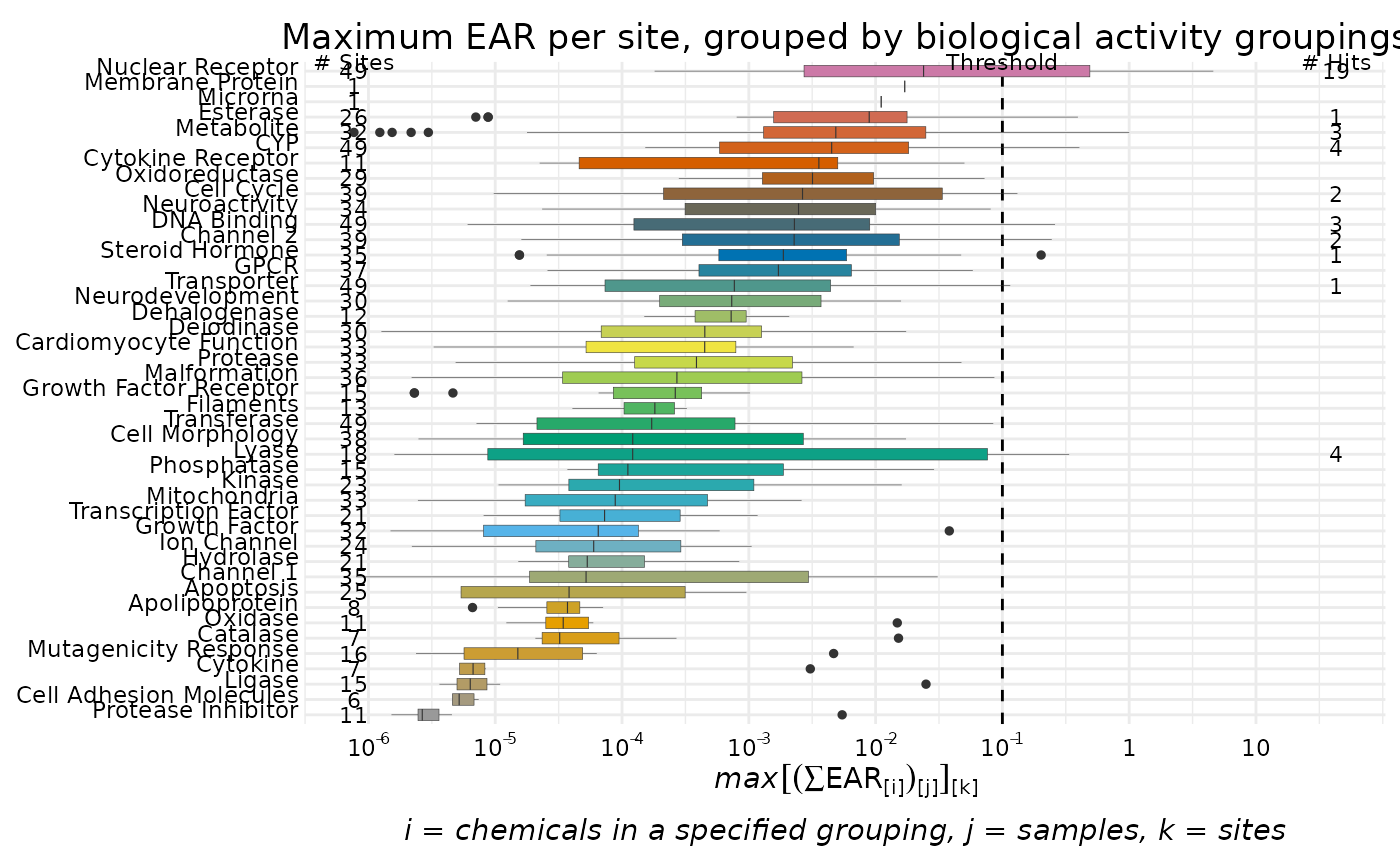plot_tox_boxplots(chemical_summary,
category = "Chemical", x_label = "EAR"
)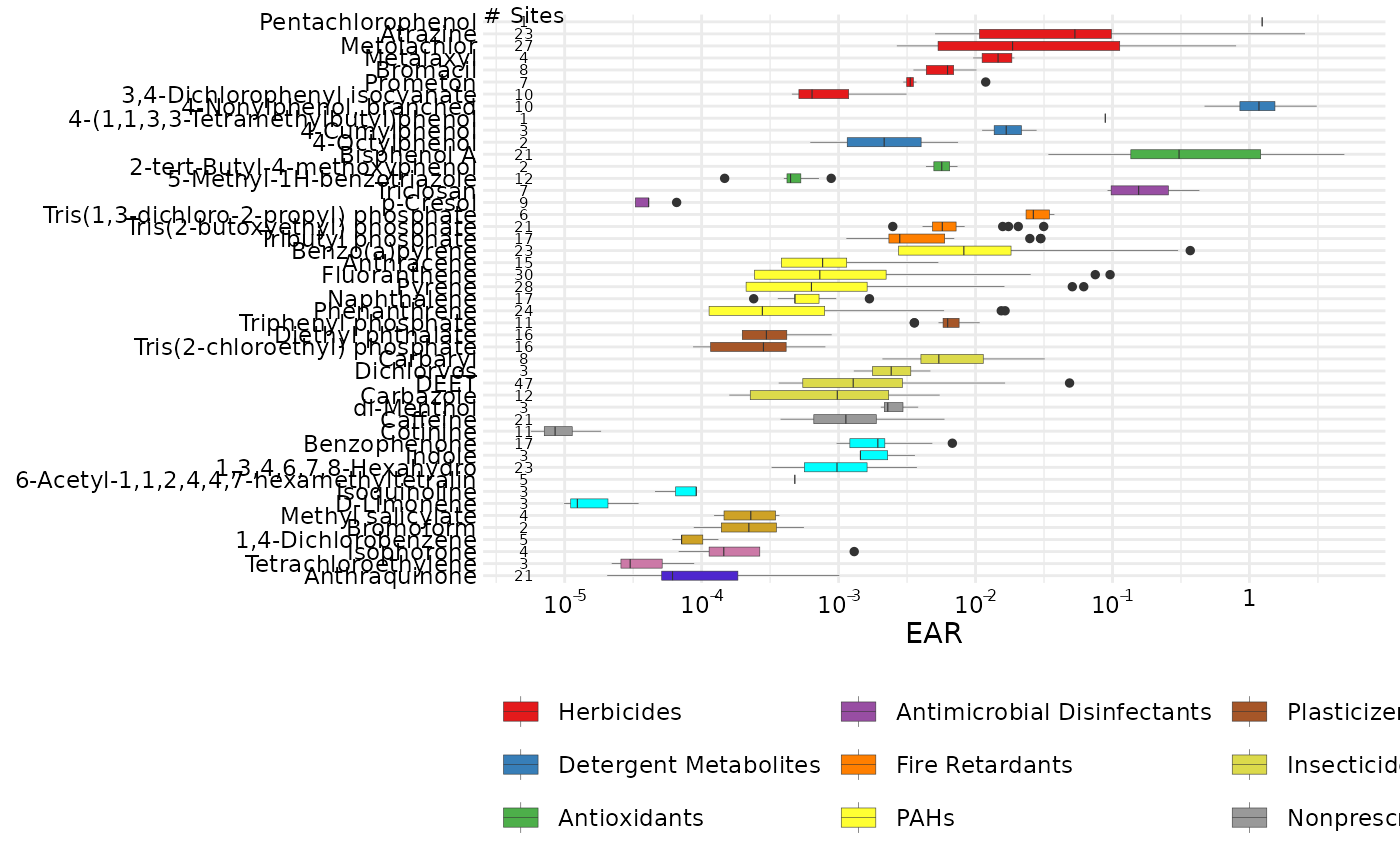single_site <- dplyr::filter(chemical_summary, site == "USGS-04024000")
plot_tox_boxplots(single_site,
category = "Biological"
)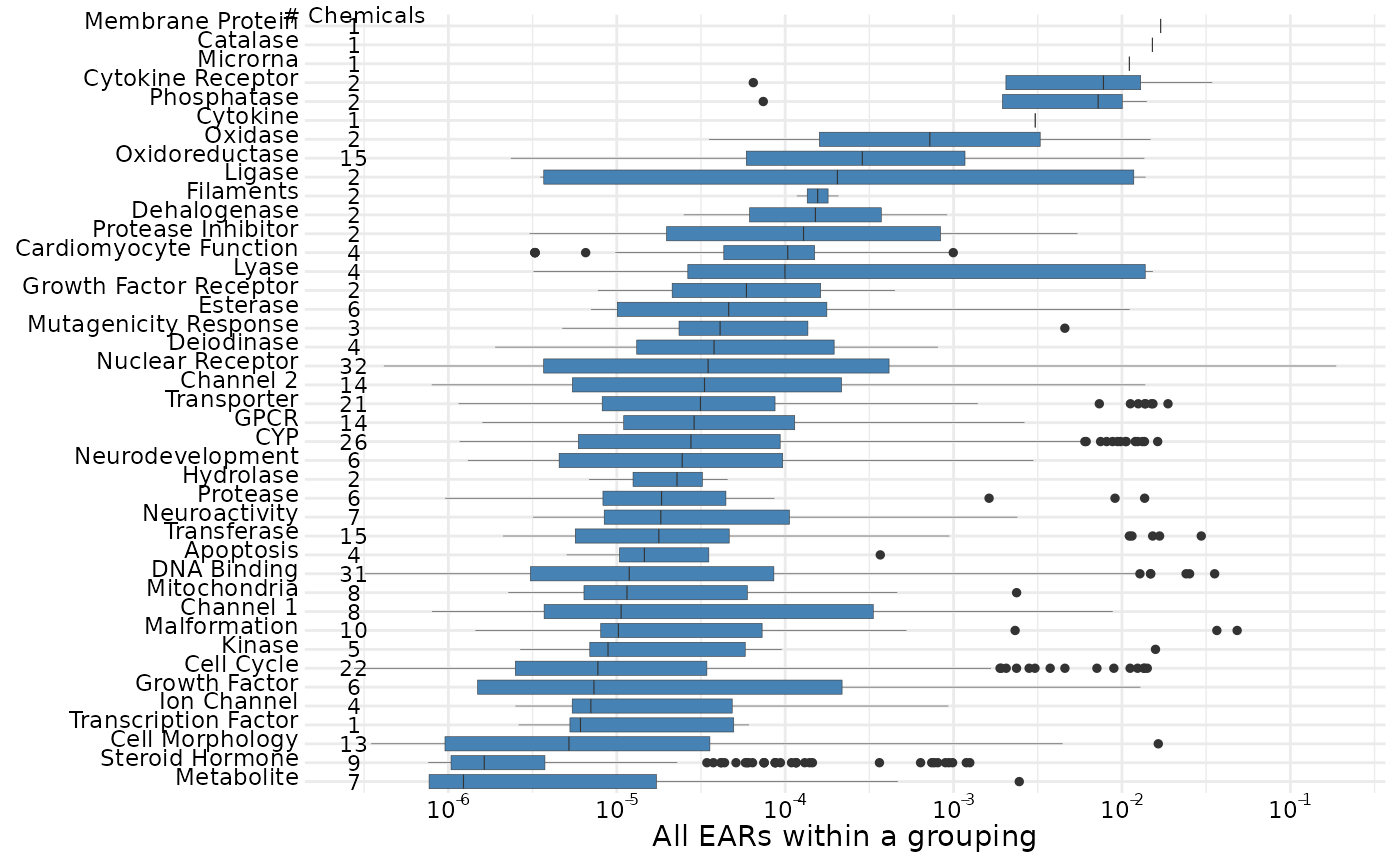plot_tox_boxplots(single_site,
category = "Chemical", hit_threshold = 0.001
)
#> Warning: Transformation introduced infinite values in continuous y-axis
#> Warning: Transformation introduced infinite values in continuous y-axis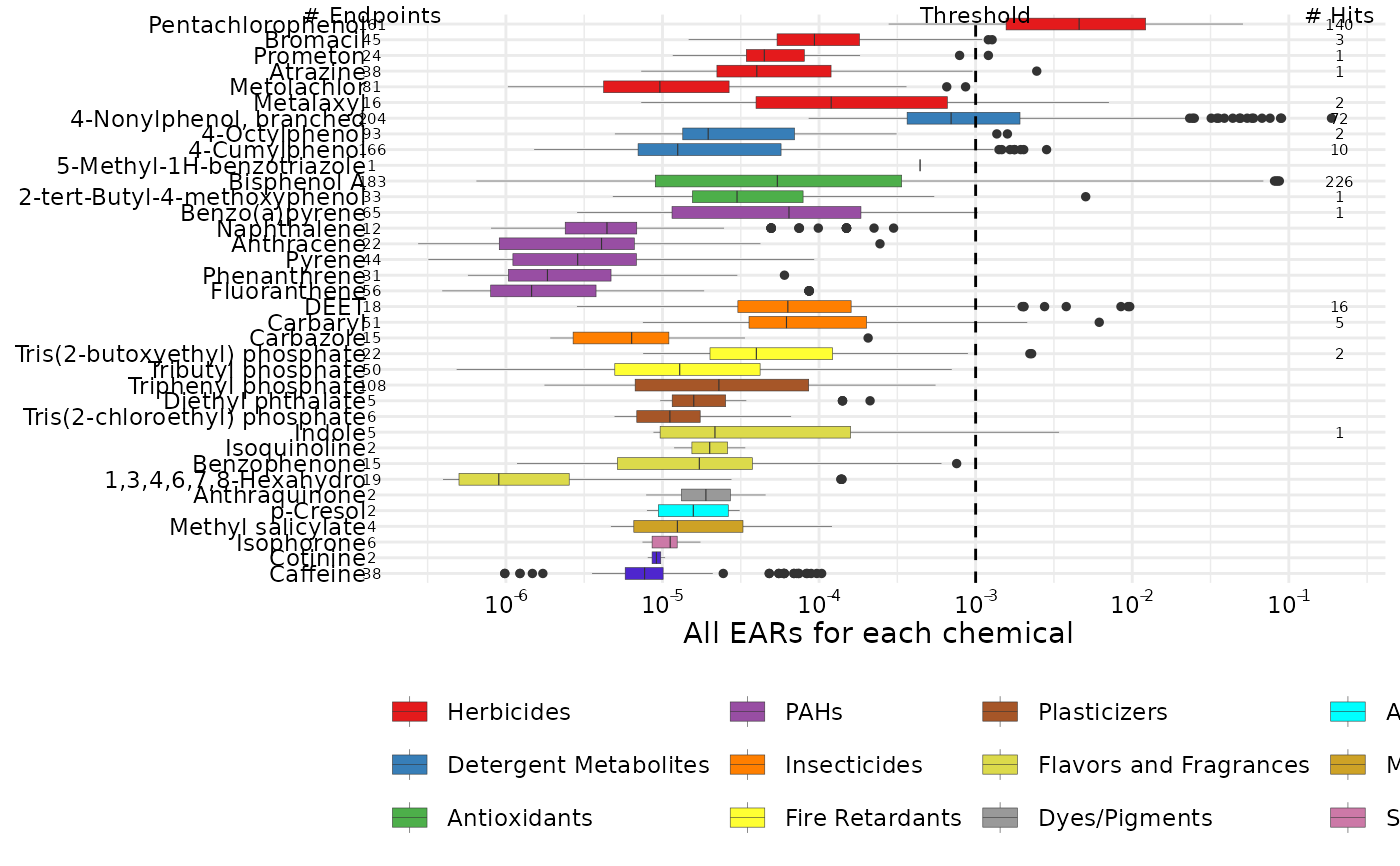# }